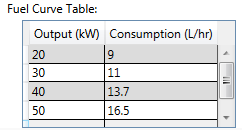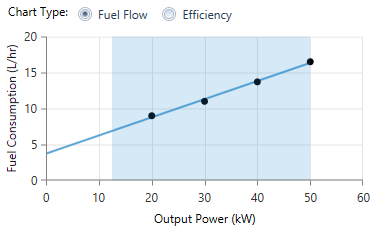# HOMER Pro 3.9

 Navigation: Design > Components Tab > Generator Fuel CurveThe Fuel Curve tab provides assistance in calculating the two fuel curve inputs on the Generator page.

## Reference Generator Capacity

Enter the rated size of the generator for which you have fuel consumption data. This input affects the value calculated for the Intercept Coefficient.

## Fuel Consumption Data

You enter data points on the generator's fuel curve in the Fuel Curve Table. You must enter at least two points.Note: The units of the fuel consumption column change according to the units of the fuel this generator uses. If the generator consumes a fuel denominated in liters, the units of the fuel consumption column will be L/hr. If the fuel is denominated in cubic meters, the units of the fuel consumption will be m3/hr.

HOMER plots the fuel consumption data in the fuel curve. The example below corresponds to the data in the table above. HOMER fits a line to the data points using the linear least-squares method. The straight line represents the line of best fit, which in this example fits the data very well. A straight line may not represent certain types of generators, such as fuel cells or variable-speed diesels, quite as well. For the more common, constant-speed internal combustion generators and microturbines, the straight-line fuel curve is a good fit.The y-intercept of the fuel curve is sometimes called the "no-load fuel consumption." This represents the amount of fuel consumed by the generator when idling (producing no electricity). The slope of the fuel curve is sometimes called the "marginal fuel consumption."

Using the straight line it fits to the fuel consumption data, HOMER calculates the generator's efficiency at various points between zero output and rated output. That calculation takes into account the energy content of the fuel. HOMER plots the results as the efficiency curve.

## Calculated Fuel Curve Parameters

Note that HOMER's two fuel curve inputs are not the intercept and slope, but rather the intercept coefficient and the slope. The intercept coefficient is equal to the intercept divided by the generator capacity. Defining the fuel curve in this manner allows HOMER to apply it to a family of generators, over a range of sizes. This is necessary when you enter multiple sizes in the "Sizes to consider" table of the Generator Inputs page because the fuel curve inputs apply to each specified generator size.

The units of the two fuel curve parameters correspond to the units of the fuel used by the generator. For example, if the fuel is measured in liters, the fuel curve slope and intercept coefficient are in units of L/hr/kW (liters per hour per kilowatt, or equivalently L/kWh).

When you click OK, HOMER copies the two calculated parameters to the Generator Inputs window.